# Multiplication

If there is one way to become better at maths, then the following is it:

YOU SHOULD GET YOUR PARENTS TO DRIVE YOU NUTS

AND TEST YOU ON THESE KEY MULTIPLICATIONS

 6times6 = 36 6times7 = 42 6times8 = 48 6times9 = 54 7times7 = 49 7times8 = 56 7times9 = 63 8times8 = 64 8times9 = 72 9times9 = 81

## When?

ALL THE TIME!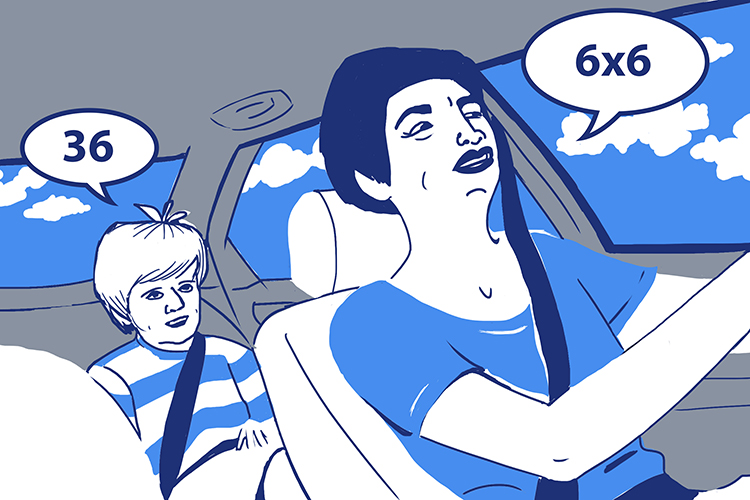In the car…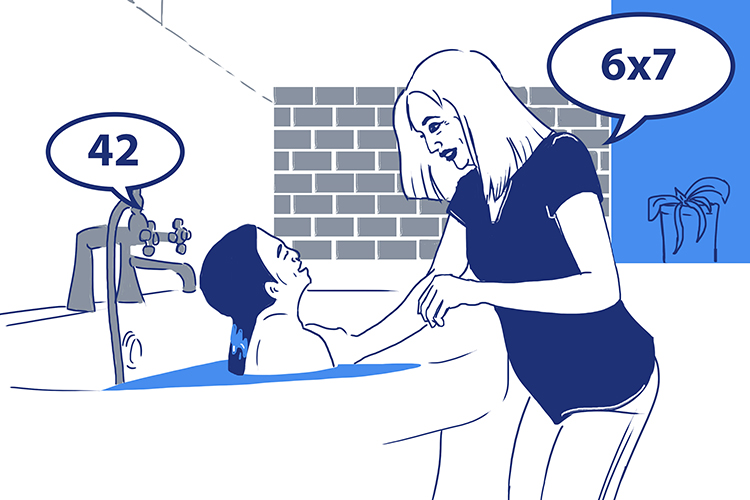In the bath…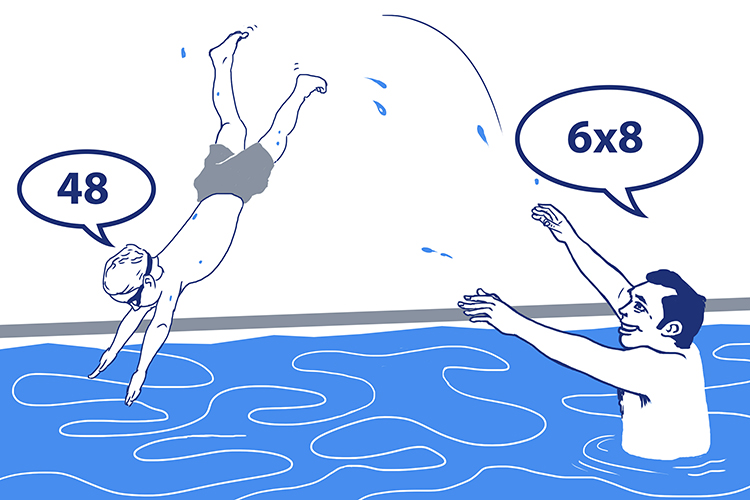In the pool…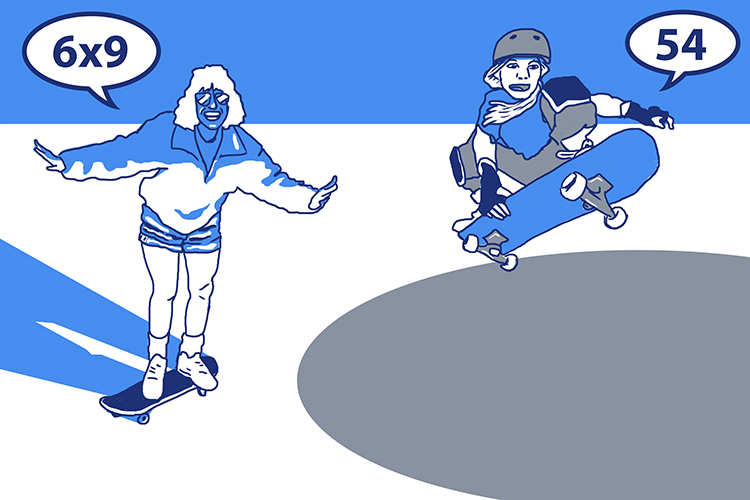On a skateboard…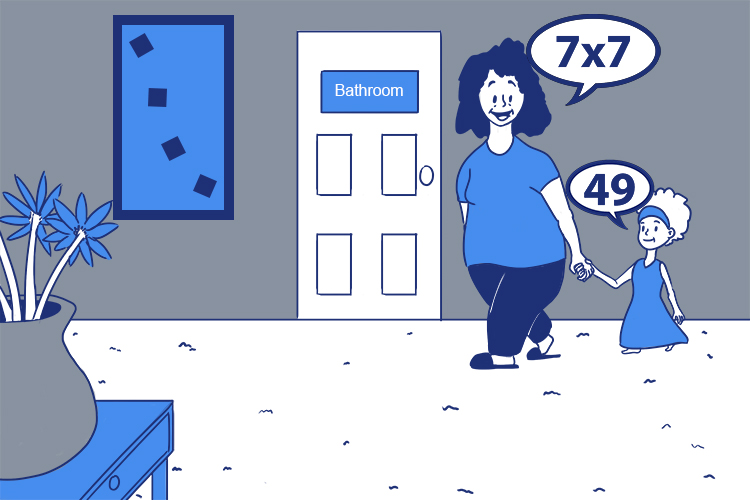In the hall…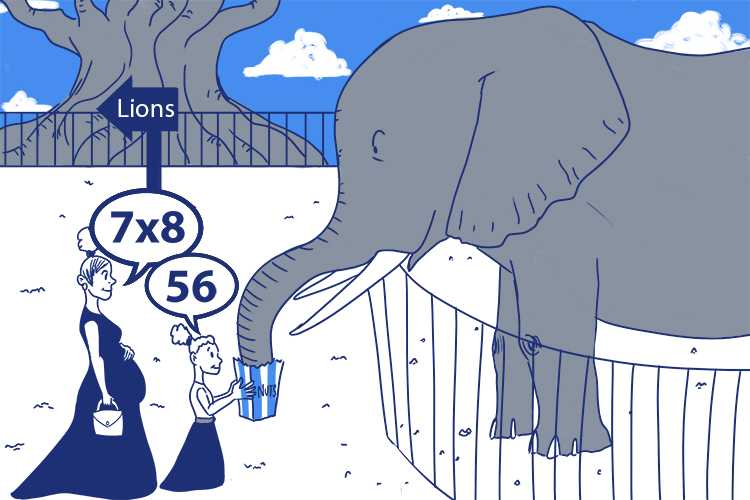In the zoo…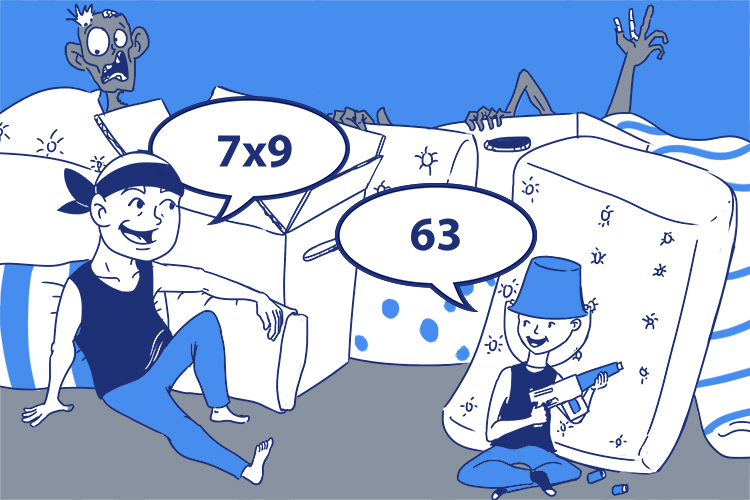In a zombie proof fort…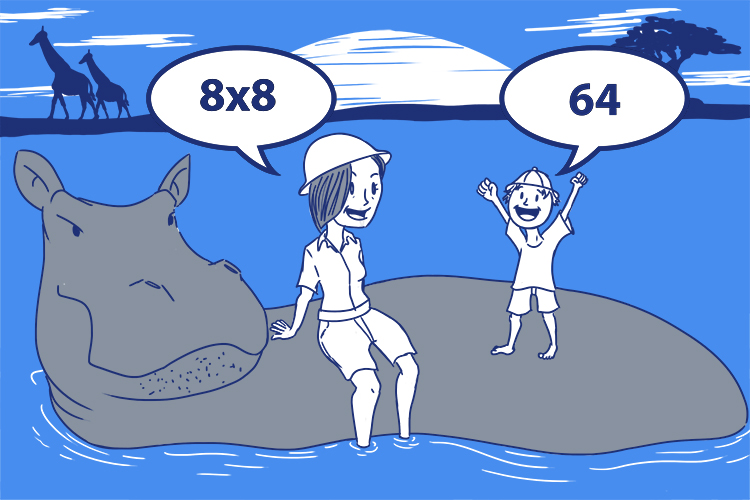On a hippo…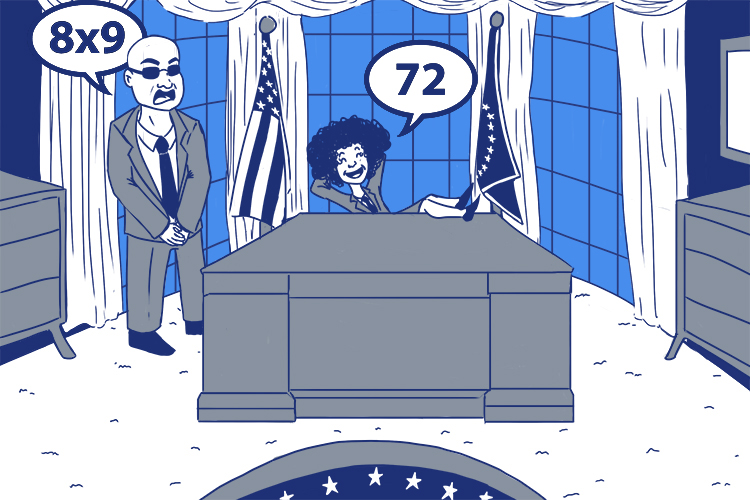In the oval office…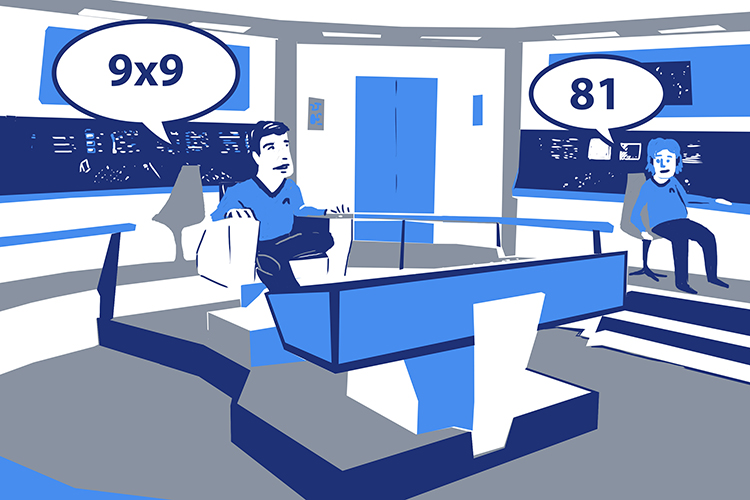On the Starship Enterprise…

SERIOUSLY – ALL THE TIME!

Those numbers again are:

 6times6 = 36 6times7 = 42 6times8 = 48 6times9 = 54 7times7 = 49 7times8 = 56 7times9 = 63 8times8 = 64 8times9 = 72 9times9 = 81

OR you could work it out as on the next sheets, but you will never be as good as in this shock treatment.